Next: Conclusion Up: wfj08w Previous: Introduction

# Results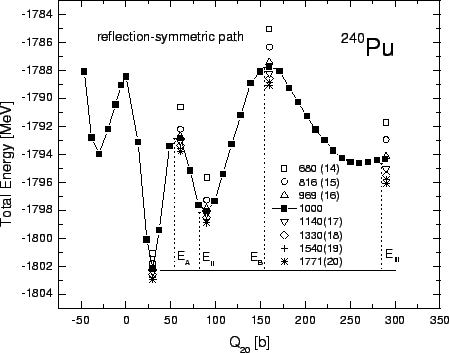First, we have studied the stability of our results with respect to the number of the deformed HO states (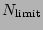) used in this basis. Figure 1 shows the calculated self-consistent potential energy curve of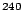Pu as a function of the axial quadrupole constraint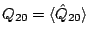. The solid line corresponds to=1000 deformed HO states included in the basis. At each value of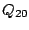, the HO basis deformation is determined as that corresponding tothrough the standard first-order expression. Therefore, the set of HO states included in the basis changes with the changing nuclear shape. The results with different values of=680 (14 spherical oscillator shells included at=0), 816 (15), 969 (16), 1140 (17), 1330 (18), 1540 (19), and 1771 (20) are also shown at the extreme points (minima and maxima) of the potential energy curve. It is seen that the rate of convergence weakly depends on the quadrupole deformation, and for larger elongations is slightly slower than at sphericity. As shown in Fig. 1, reliable calculations can be carried out with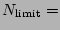1140 (17), and the errors related to the finite basis sizes that impact energies of the first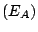and second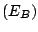barrier are less than 1 MeV.

Having determined the basis size, we performed the set of calculations for the series of even-even Fermium isotopes which are experimentally known to exhibit rapid variations of the spontaneous fission half-lives. Two fission paths corresponding to bimodal fission have been investigated. The usual reflection-asymmetric path corresponds to two fission fragments with different masses. The reflection-symmetric path can be associated with division into symmetric, nearly spherical fragments with high kinetic energies. In our calculations, the quadrupole and octupole mass moments were used as constraints. The non-zero octupole mass moment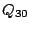indicates the breaking of intrinsic parity, while the presence of the quadrupole moment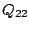signals the appearance of triaxial distortions.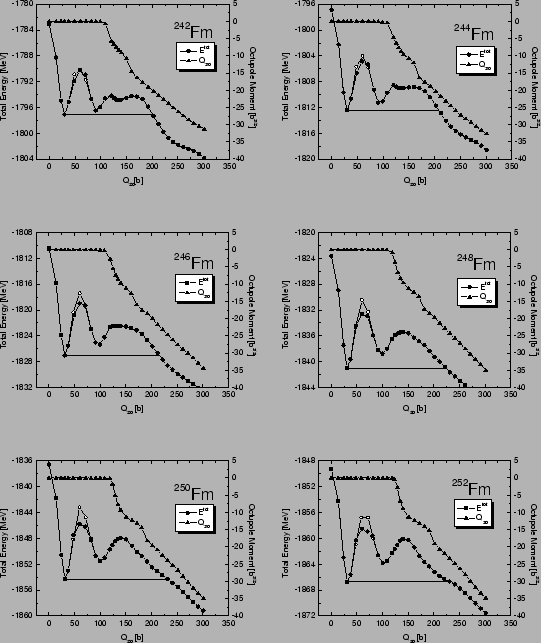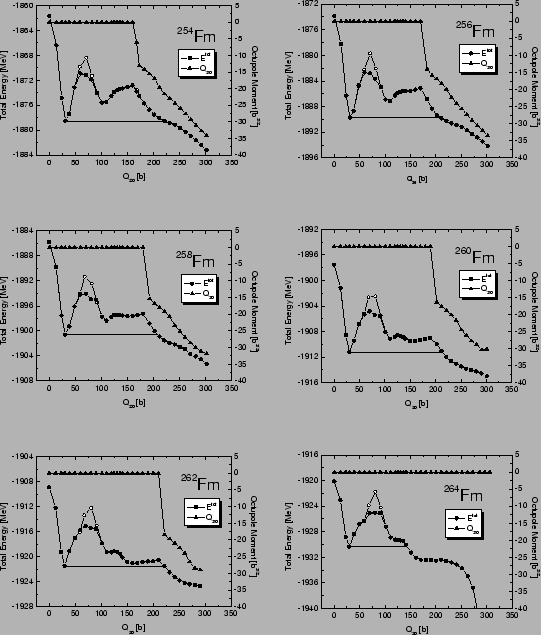Deformation energy curves (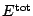) and the mass octupole moments () calculated along static fission paths for the even-even Fermium isotopes with the neutron numbers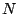=142-164 are plotted in Figs. 2 and 3 as functions of the mass quadrupole moment. All calculated static barriers have similar, two-humped shapes. The sizes of the static fission barriers are correlated with the experimentally known increase in the spontaneous fission half-lives for the lighter Fermium isotopes with=142-152. The influence of triaxial asymmetry on the height of the first fission barrier is shown as a difference between the open and solid symbols. The effect of triaxiality increases with neutron number and reaches about 3MeV for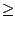150. For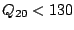b, the fission paths of nuclei displayed in Fig. 2 exhibit reflection-symmetric shapes (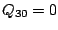), while the reflection-asymmetric paths (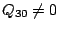) takes over at larger elongations with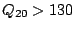b. For the heavier Fm isotopes displayed in Fig. 3, the transition to the reflection-asymmetric path occurs at greater values ofand the transition point increases with. In the extreme case of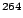Fm (which is expected to fission into two doubly magic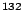Sn nuclei), the fission path becomes reflection-symmetric. The disappearance of the reflection-asymmetric path at large neutron numbers gives rise to the reduction, or even disappearance, of the second fission barrier. This reduction plays a crucial role in the standard interpretation of the experimentally known rapid decrease of the spontaneous fission half-lives in the heavy Fm isotopes.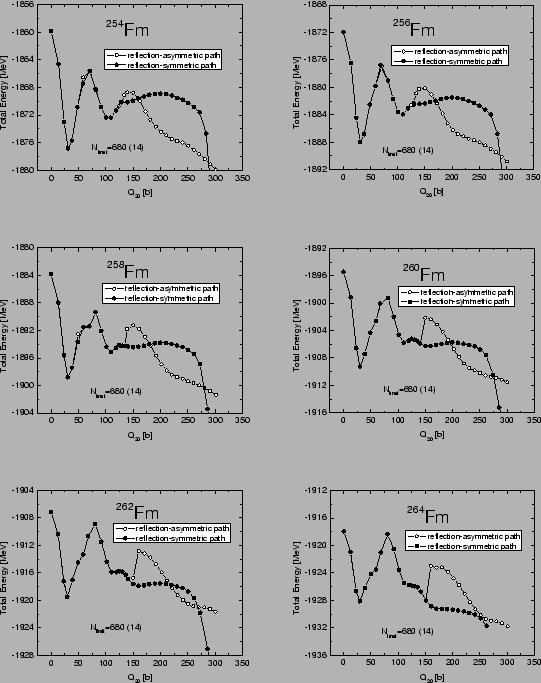In order to better understand the competition between reflection-symmetric and reflection-asymmetric fission valleys, we show in Fig. 4 the total energy curves for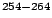Fm calculated with a smaller basis=680. For each nucleus the two different fission paths are displayed: reflection-asymmetric (open symbols) and reflection-symmetric (solid symbols). It is seen that at large values of, the barriers along the reflection-asymmetric paths are more narrow, whereas the distinctly higher second humps appear.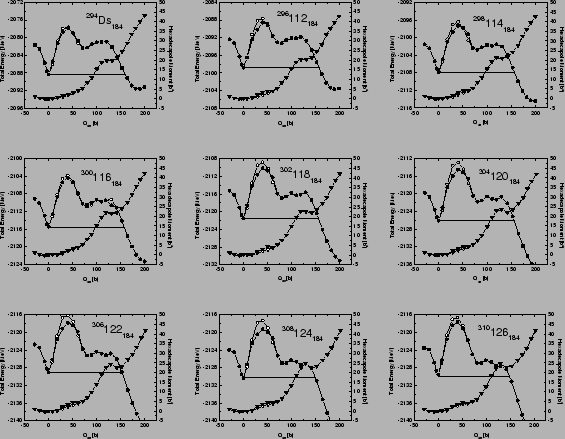Figure 5 shows the deformation energy curves and the mass hexadecapole moments calculated along the static fission paths of the superheavy even-even nuclei with=184 (which is the neutron magic gap in this region). These results markedly differ from those obtained for the Fm isotopes. As expected, all=184 isotones have spherical ground states. The corresponding static two-humped fission barriers are narrow and reflection-symmetric. The triaxial deformation lowers the first barrier, with the maximum reduction (2 MeV) predicted for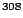124. As illustrated in Fig. 5, with increasing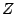, the height of the first barrier increases while the second barrier gets reduced. The increase in the hexadecapole moment along the fission path reflects the development of the neck.Next: Conclusion Up: wfj08w Previous: Introduction
Jacek Dobaczewski 2005-01-23